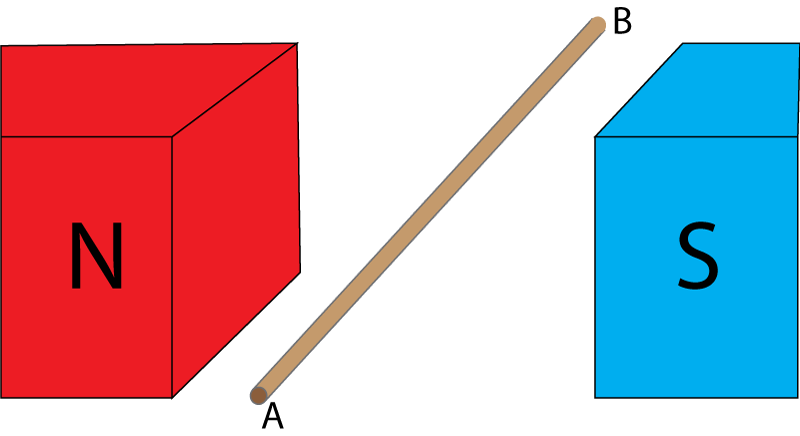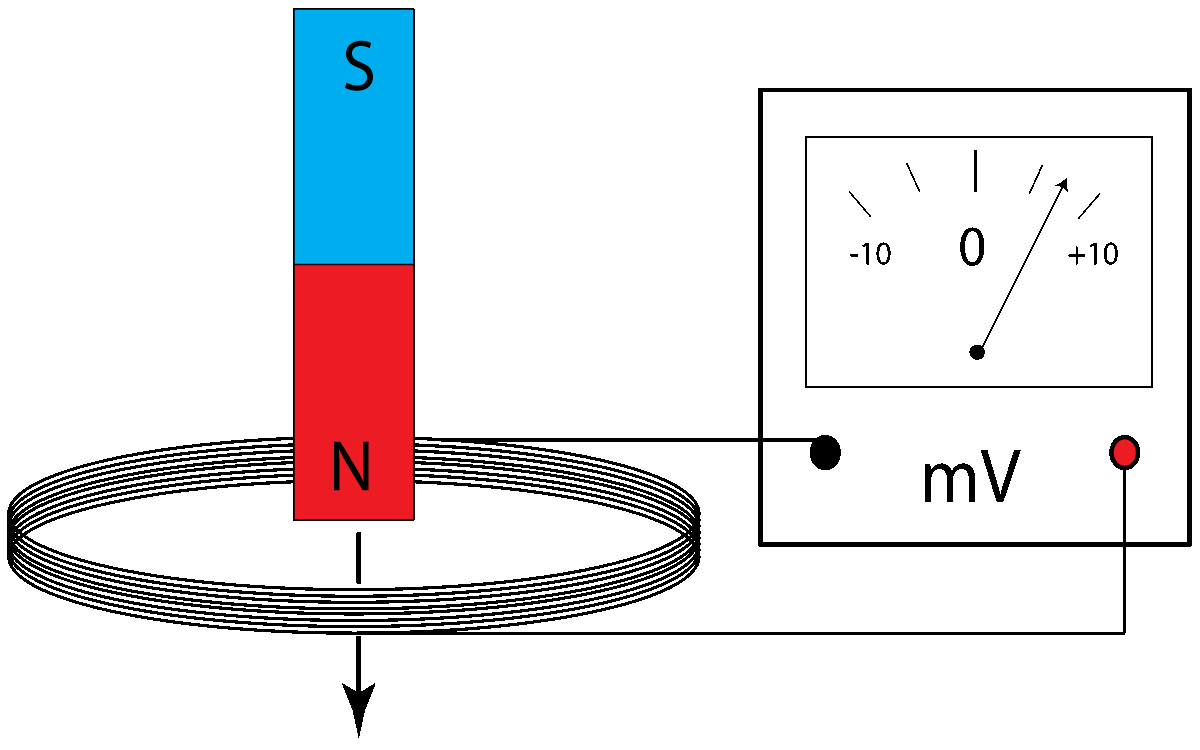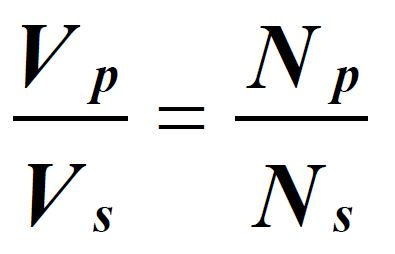Induction and Transformers

1. What does 'induction' mean?

• A. The production of a force on a conductor due to a magnetic field.
• B. The production of a force on a conductor due to friction.
• C. The production of a voltage in a conductor due to a magnetic field.
• D. The production of a voltage in a conductor due to friction.

2. The image shows an aluminium rod placed between 2 magnets.How can a voltage be induced in this rod between the ends labelled A and B?

• A. Replace the north pole with another south pole.
• B. Change the aluminium rod for a copper rod.
• C. Move the rod upwards or downwards.
• D. Heat the rod.

3&4. A magnet is dropped through a coil connected to a millivoltmeter as shown. The voltmeter needle moves to the right showing a positve reading.3. Which of these will make the voltmeter reading smaller but still positive?

• A). Drop the south pole through first.
• B). Add more turns to the coil.
• C). Move the magnet slowly through the coil.
• D). Use a stronger magnet.

4. Which of these will make the voltmeter move to the left?

• A). Drop the south pole through first.
• B). Add more turns to the coil.
• C). Move the magnet slowly through the coil.
• D). Use a stronger magnet.

5. The diagram shows an a.c. generator - a coil is rotated between two magnets to produce a voltage to heat a resistor, labelled the load in the diagram.Which of these sentences here best describes the changes that take place if the coil is rotated faster?

• A. The alternating voltage across the resistor and the frequency both remain constant.
• B. The alternating voltage across the resistor remains constant, but the frequency of the a.c. increases.
• C. The alternating voltage across the resistor increases and the frequency also increases.
• D. The alternating voltage across the resistor increases but the frequency stays the same.
6. A device that uses sound waves to move a coil can induce a small voltage signal in an external circuit that represents the sound. It is converting the energy in sound waves into electrcial energy.

What is the name given to this device?

• A. A loudspeaker.
• B. A dynamo.
• C. A generator.
• D. A microphone.

7-9. A transformer is used to increase or decrease the voltage for an appliance.

What are the missing values in the table below?

Transformer formula:Input Voltage (V) Number of turns on primary number of turns on secondary output voltage (V) 7. 20 100 500 ? 100 1000 4 40 8. 12 ? 80 800 3200 64000 1600 240 9. 12 400 ? 80 800 200 2000 60

10. The national grid uses step up transformers to produce very high voltages in long cables that link towns and cities. Why is this?

• A. People are less likely to steal the electrcial energy because it is dangerously high voltage.
• B. The transformers step up the power delivered by the same ratio as the step up in voltage.
• C. Houses and offices need this very high voltage to operate.
• D. Less current is needed, and therefore there is less power loss in the cables.